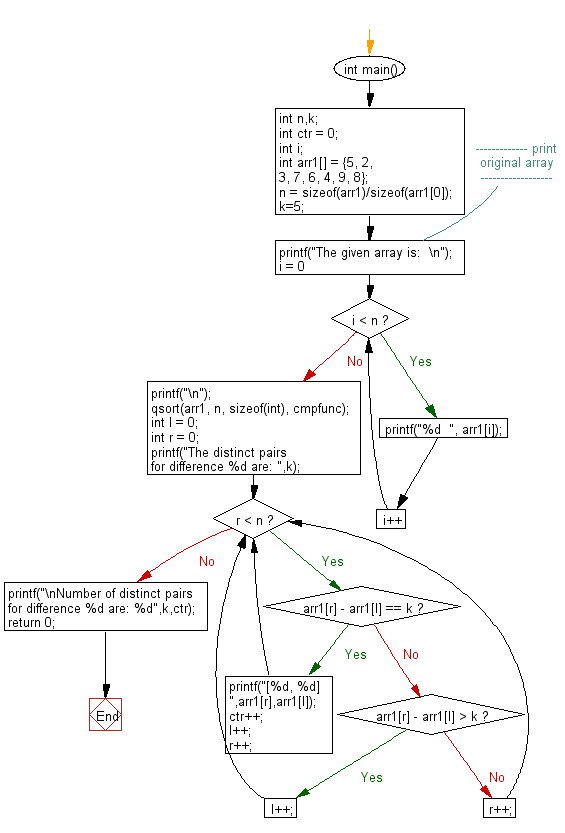﻿ C exercises: Count all distinct pairs for a specific difference - w3resource# C Exercises: Count all distinct pairs for a specific difference

## C Array: Exercise-80 with Solution

Write a program in C to count all distinct pairs for a specific difference.

Sample Solution:

C Code:

``````#include <stdio.h>
#include <stdlib.h>
#include <stdbool.h>
#define MAX 1000000
int cmpfunc (const void * arr1, const void * b)
{
return ( *(int*)arr1 - *(int*)b );
}
int main()
{
int n,k;
int ctr = 0;
int i;
int arr1[] = {5, 2, 3, 7, 6, 4, 9, 8};
n = sizeof(arr1)/sizeof(arr1);
k=5;
//------------- print original array ------------------
printf("The given array is:  \n");
for(i = 0; i < n; i++)
{
printf("%d  ", arr1[i]);
}
printf("\n");
//------------------------------------------------------
qsort(arr1, n, sizeof(int), cmpfunc);
int l = 0;
int r = 0;
printf("The distinct pairs for difference %d are: ",k);
while(r < n)
{
if(arr1[r] - arr1[l] == k)
{
printf("[%d, %d] ",arr1[r],arr1[l]);
ctr++;
l++;
r++;
}
else if(arr1[r] - arr1[l] > k)
l++;
else
r++;
}
printf("\nNumber of distinct pairs for difference %d are: %d",k,ctr);
return 0;
}
```
```

Sample Output:

```The given array is:
5  2  3  7  6  4  9  8
The distinct pairs for difference 5 are: [7, 2] [8, 3] [9, 4]
Number of distinct pairs for difference 5 are: 3
```

Pictorial Presentation:Flowchart:C Programming Code Editor:

Improve this sample solution and post your code through Disqus.

What is the difficulty level of this exercise?

﻿

## C Programming: Tips of the Day

Where is the C auto keyword used?

auto is a modifier like static. It defines the storage class of a variable. However, since the default for local variables is auto, you don't normally need to manually specify it.

Ref : https://bit.ly/3yzwC9r